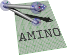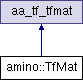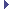amino  1.0-beta2 Lightweight Robot Utility Library
amino::TfMat Struct Reference

A transformation matrix object. More...

#include <tf.hpp>

Inheritance diagram for amino::TfMat:## Public Member Functions

TfMat ()
Construct an identity transformation matrix.

TfMat (const struct aa_tf_tfmat *T)
Construct a transformation matrix from another transformation matrix.

TfMat (const struct aa_tf_tfmat &T)
Construct a transformation matrix from another transformation matrix.

TfMat (const struct aa_tf_duqu *S)
Construct a transformation matrix from a dual quaternion.

TfMat (const struct aa_tf_duqu &S)
Construct a transformation matrix from a dual quaternion.

TfMat (const struct aa_tf_qv *S)
Construct a transformation matrix from a quaternion-translation.

TfMat (const struct aa_tf_qv &S)
Construct a transformation matrix from a quaternion-translation.

TfMat (const struct aa_tf_quat *_r, const struct aa_tf_vec3 *_v)
Construct a transformation matrix from a quaternion-translation.

TfMat (const struct aa_tf_quat &_r, const struct aa_tf_vec3 &_v)
Construct a transformation matrix from a quaternion-translation.

## Static Public Member Functions

static aa_tf_tfmat from_duqu (const double s)
Construct a transformation matrix from a dual quaternion.

static aa_tf_tfmat from_qv (const double q, const double v)
Construct a transformation matrix from a rotation quaternion and translation vector.

static aa_tf_tfmat from_tfmat (const double t)
Construct a transformation matrix from another transformation matrix.

## Additional Inherited MembersPublic Attributes inherited from aa_tf_tfmat
union {
struct {
double   R 
the rotation matrix part

aa_tf_vec3_t   v
the origin vector part

}

double   data 
data array

};

## Detailed Description

A transformation matrix object.

Definition at line 910 of file tf.hpp.

The documentation for this struct was generated from the following file: